Next: Lagrange brackets Up: Derivation of Lagrange planetary Previous: Introduction

# Preliminary analysis

According to Equation (G.6), we have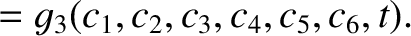(G.13)

If this expression, and the analogous expressions for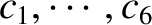and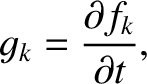, were differentiated with respect to time, and the results substituted into Equations (G.3)-(G.5), then we would obtain three time evolution equations for the six variables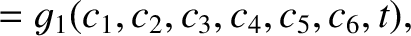. In order to make the problem definite, three additional conditions must be introduced into the problem. It is convenient to choose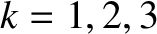(G.14)

for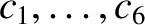. Hence, it follows from Equations (G.12) and (G.13) that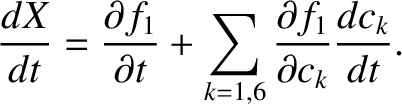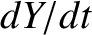(G.15)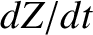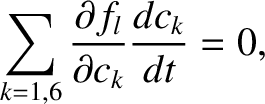(G.16) and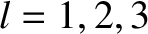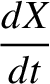(G.17)

Differentiation of these equations with respect to time yields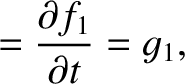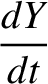(G.18)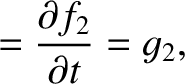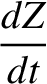(G.19) and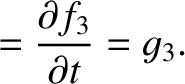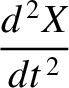(G.20)

Substitution into Equations (G.3)-(G.5) gives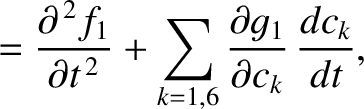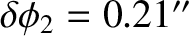(G.21)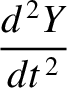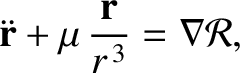(G.22) and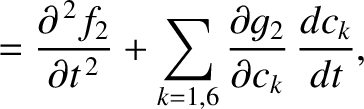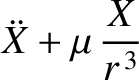(G.23)

where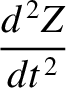. Because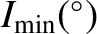,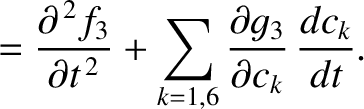, and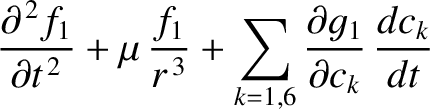are the respective solutions to Equation (G.3)-(G.5) when the right-hand sides are zero, and the orbital elements are thus constants, it follows that the first two terms in each of the preceding three equations cancel one another. Hence, writingas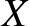, and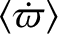as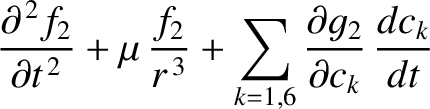, and so on, Equations (G.14) and (G.21)-(G.23) yield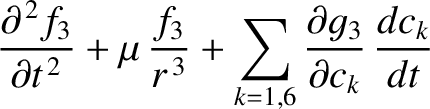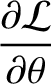(G.24)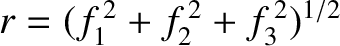(G.25)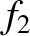(G.26)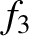(G.27)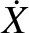(G.28) and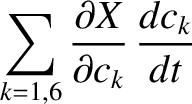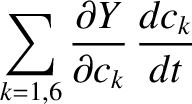(G.29)

These six equations are equivalent to the three original equations of motion [(G.3)-(G.5)].Next: Lagrange brackets Up: Derivation of Lagrange planetary Previous: Introduction
Richard Fitzpatrick 2016-03-31